# 6 0476 878 Computational Biology Genomes Networks Evolution

• Slides: 36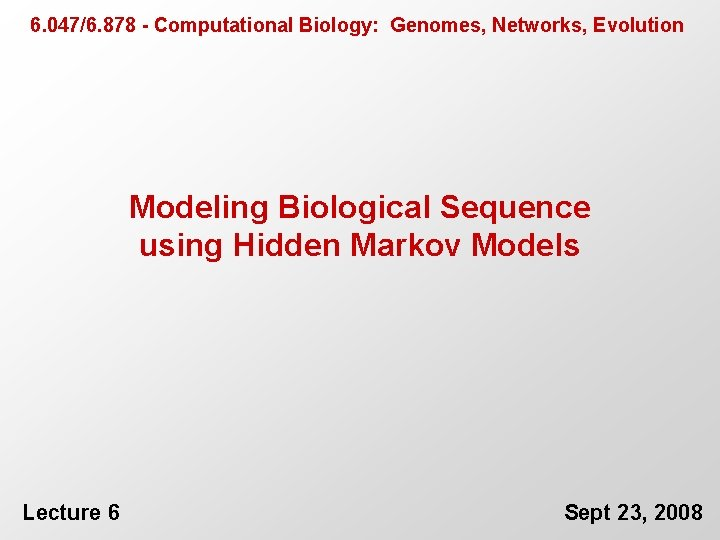6. 047/6. 878 - Computational Biology: Genomes, Networks, Evolution Modeling Biological Sequence using Hidden Markov Models Lecture 6 Sept 23, 2008Challenges in Computational Biology 4 Genome Assembly 9 Regulatory motif discovery 6 Gene Finding DNA 2 Sequence alignment 14 Comparative Genomics 10 Evolutionary Theory 5 TCATGCTAT TCGTGATAA TGAGGATAT TTATCATAT TTATGATTT 3 Database search Gene expression analysis RNA transcript 4 Cluster discovery 11 Protein network analysis 12 Regulatory network inference 13 Emerging network properties 9 Gibbs samplingCourse Outline Lec 1 2 3 4 5 6 Date Thu Sep 04 Tue Sep 09 Thu Sep 11 Tue Sep 16 Thu Sep 18 Tue Sep 23 Topic Intro: Biology, Algorithms, Machine Learning Recitation 1: Probability, Statistics, Biology Global/local alignment/Dyn. Prog String. Search/Blast/DB Search Recitation 2: Affine gaps alignment / Hashing with combs Clustering/Basics/Gene Expression/Sequence Clustering Classification/Feature Selection/ROC/SVM Recitation 3: Microarrays HMMs 1 - Evaluation / Parsing Homework PS 1 (due 9/15) PS 2 (due 9/22) 7 Thu Sep 25 Tue Sep 30 Thu Oct 02 Tue Oct 07 Thu Oct 09 Tue Oct 14 Thu Oct 16 Tue Oct 21 Thu Oct 23 Tue Oct 28 Thu Oct 30 Tue Nov 04 Thu Nov 06 Tue Nov 11 Thu Nov 13 Tue Nov 18 Thu Nov 20 Tue Nov 25 Thu Nov 27 Tue Dec 02 Thu Dec 04 Tue Dec 09 HMMs 2 - Posterior. Decoding/Learning Recitation 4: Posterior decoding review, Baum-Welch Learning Generalized HMMs and Gene Prediction Regulatory Motifs/Gibbs Sampling/EM Recitation 5: Entropy, Information, Background models Gene evolution: Phylogenetic algorithms, NJ, ML, Parsimony Molecular. Evolution/Coalescence/Selection/MKS/Ka. Ks Recitation 6: Gene Trees, Species Trees, Reconciliation Population Genomics - Fundamentals Population Genomics - Association Studies Recitation 7: Population genomics MIDTERM (Review Session Mon Oct 20 rd at 7 pm) Genome. Assembly/Euler. Graphs Recitation 8: Brainstorming for Final Projects (MIT) Comparative. Genomics 1: Biological. Signal. Discovery/Evolutionary. Signatures Comparative. Genomics 2: Phylogenomics, Gene and Genome Duplication Conditional Random Fields/Gene Finding/Feature Finding Regulatory Networks/Bayesian Networks Veterans Day Holiday Inferring Biological Networks/Graph Isomorphism/Network Motifs Metabolic Modeling 1 - Dynamic systems modeling Metabolic Modeling 2 - Flux balance analysis & metabolic control analysis Systems Biology (Uri Alon) Thanks. Giving Break Module Networks (Aviv Regev) Synthetic Biology (Tom Knight) Final Presentations - Part I (11 am) Final Presentations - Part II (5 pm) PS 3 (due 10/06) PS 4 (due 10/20) Midterm Project Phase I (due 11/06) Project Phase II (due 11/24) Project Phase III (due 12/08) 8 9 10 11 12 13 14 15 16 17 18 19 20 21 22 23 24 25 26 27What have we learned so far? • String searching and counting – Brute-force algorithm – W-mer indexing • Sequence alignment – Dynamic programming, duality path alignment – Global / local alignment, general gap penalties • String comparison – Exact string match, semi-numerical matching • Rapid database search – Exact matching: Hashing, BLAST – Inexact matching: neighborhood search, projections • Problem set 1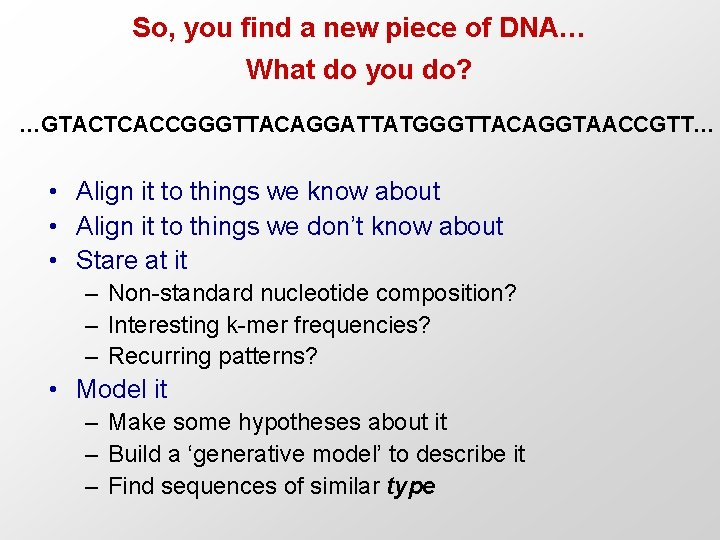So, you find a new piece of DNA… What do you do? …GTACTCACCGGGTTACAGGATTATGGGTTACAGGTAACCGTT… • Align it to things we know about • Align it to things we don’t know about • Stare at it – Non-standard nucleotide composition? – Interesting k-mer frequencies? – Recurring patterns? • Model it – Make some hypotheses about it – Build a ‘generative model’ to describe it – Find sequences of similar type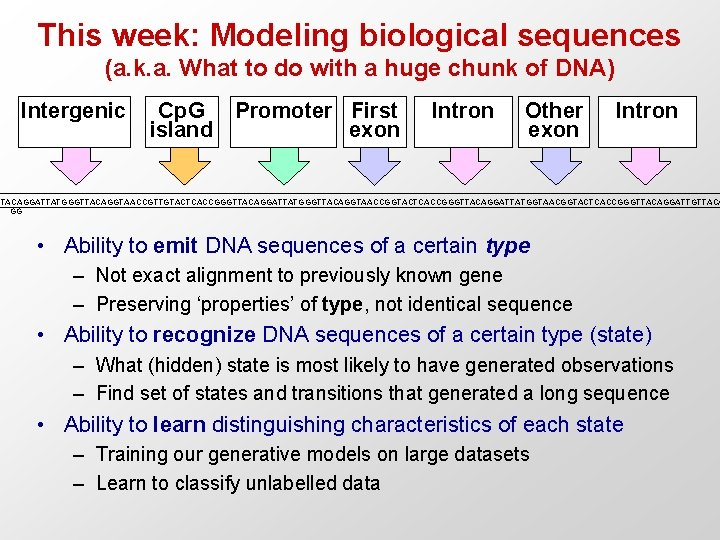This week: Modeling biological sequences (a. k. a. What to do with a huge chunk of DNA) Intergenic Cp. G Promoter First island exon Intron Other exon Intron TTACAGGATTATGGGTTACAGGTAACCGTTGTACTCACCGGGTTACAGGATTATGGGTTACAGGTAACCGGTACTCACCGGGTTACAGGATTATGGTAACGGTACTCACCGGGTTACAGGATTGTTACA GG • Ability to emit DNA sequences of a certain type – Not exact alignment to previously known gene – Preserving ‘properties’ of type, not identical sequence • Ability to recognize DNA sequences of a certain type (state) – What (hidden) state is most likely to have generated observations – Find set of states and transitions that generated a long sequence • Ability to learn distinguishing characteristics of each state – Training our generative models on large datasets – Learn to classify unlabelled data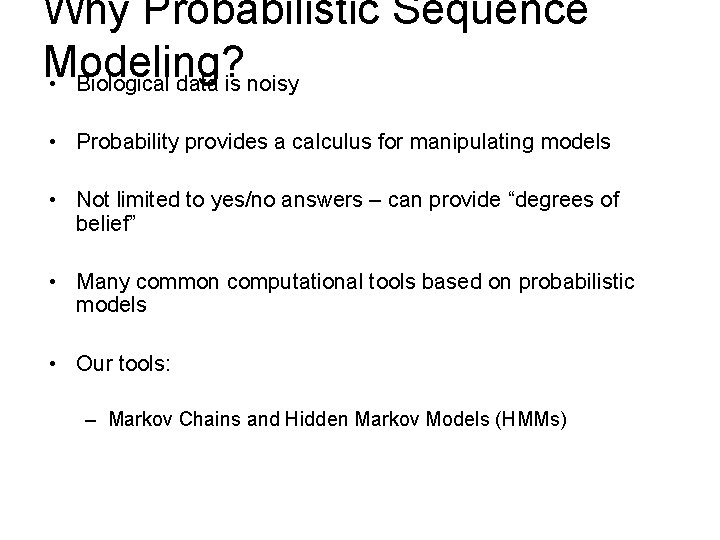Why Probabilistic Sequence Modeling? • Biological data is noisy • Probability provides a calculus for manipulating models • Not limited to yes/no answers – can provide “degrees of belief” • Many common computational tools based on probabilistic models • Our tools: – Markov Chains and Hidden Markov Models (HMMs)Definition: Markov Chain Definition: A Markov chain is a triplet (Q, p, A), where: Ø Q is a finite set of states. Each state corresponds to a symbol in the alphabet Σ Ø p is the initial state probabilities. Ø A is the state transition probabilities, denoted by ast for each s, t in Q. Ø For each s, t in Q the transition probability is: ast ≡ P(xi = t|xi-1 = s) Output: The output of the model is the set of states at each instant time => the set of states are observable Property: The probability of each symbol xi depends only on the value of the preceding symbol xi-1 : P (xi | xi-1, …, x 1) = P (xi | xi-1) Formula: The probability of the sequence: P(x) = P(x. L, x. L-1, …, x 1) = P (x. L | x. L-1) P (x. L-1 | x. L-2)… P (x 2 | x 1) P(x 1)Definitions: HMM (Hidden Markov Model) Definition: An HMM is a 5 -tuple (Q, V, p, A, E), where: Ø Q is a finite set of states, |Q|=N Ø V is a finite set of observation symbols per state, |V|=M Ø p is the initial state probabilities. Ø A is the state transition probabilities, denoted by ast for each s, t in Q. Ø For each s, t in Q the transition probability is: ast ≡ P(xi = t|xi-1 = s) Ø E is a probability emission matrix, esk ≡ P (vk at time t | qt = s) Output: Only emitted symbols are observable by the system but not the underlying random walk between states -> “hidden” Property: Emissions and transitions are dependent on the current state only and not on the past.The six algorithmic settings for HMMs Learning Decoding Scoring One path 1. Scoring x, one path P(x, π) All paths 2. Scoring x, all paths P(x) = Σπ P(x, π) Prob of a path, emissions Prob of emissions, over all paths 3. Viterbi decoding 4. Posterior decoding π* = argmaxπ P(x, π) π^ = {πi | πi=argmaxk ΣπP(πi=k|x)} Most likely path Path containing the most likely state at any time point. 5. Supervised learning, given π Λ* = argmaxΛ P(x, π|Λ) 6. Unsupervised learning. Λ* = argmaxΛ maxπP(x, π|Λ) Viterbi training, best path 6. Unsupervised learning Λ* = argmaxΛ ΣπP(x, π|Λ) Baum-Welch training, over all pathsExample 1: Finding GC-rich regions • Promoter regions frequently have higher counts of Gs and Cs • Model genome as nucleotides drawn independently from two distributions: Background (B) and Promoters (P). • Emission probabilities based on nucleotide composition in each. • Transition probabilities based on relative abundance & avg. length 0. 15 0. 85 Background (B) Promoter Region (P) 0. 75 0. 25 A: 0. 25 T: 0. 25 G: 0. 25 C: 0. 25 P(Xi|B) A: 0. 15 T: 0. 13 G: 0. 30 C: 0. 42 P(Xi|P) TAAGAATTGTGTCACATAAAAACCCTAAGTTAGAGGATTGAGATTGGCA GACGATTGTTCGTGATAATAAACAAGGGGGGCATAGATCAGGCTCATATTGGC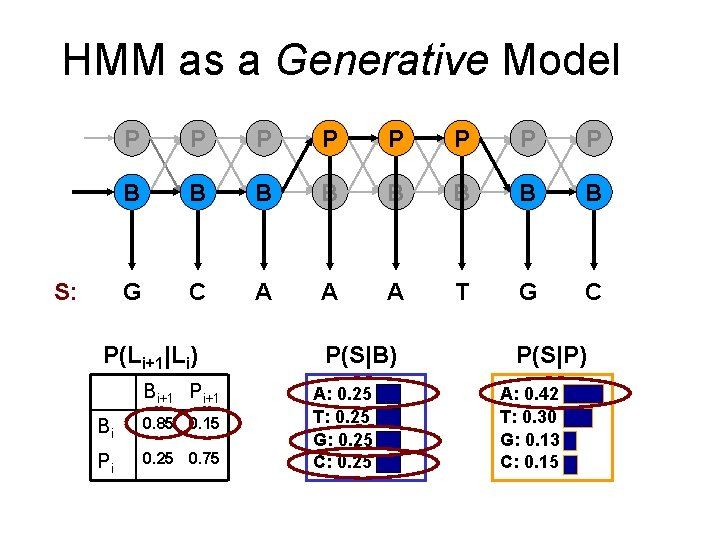HMM as a Generative Model S: P P P P B B B B G C A A A T G C P(Li+1|Li) Bi+1 Pi+1 Bi 0. 85 0. 15 Pi 0. 25 0. 75 P(S|B) A: 0. 25 T: 0. 25 G: 0. 25 C: 0. 25 P(S|P) A: 0. 42 T: 0. 30 G: 0. 13 C: 0. 15Sequence Classification PROBLEM: Given a sequence, is it a promoter region? – We can calculate P(S|MP), but what is a sufficient P value? SOLUTION: compare to a null model and calculate log-likelihood ratio – e. g. background DNA distribution model, B Pathogenicity Islands A: 0. 15 Background DNA Score Matrix A: -0. 73 A: T: 0. 13 T: -0. 94 T: G: 0. 30 G: 0. 26 C: 0. 42 C: 0. 74 C: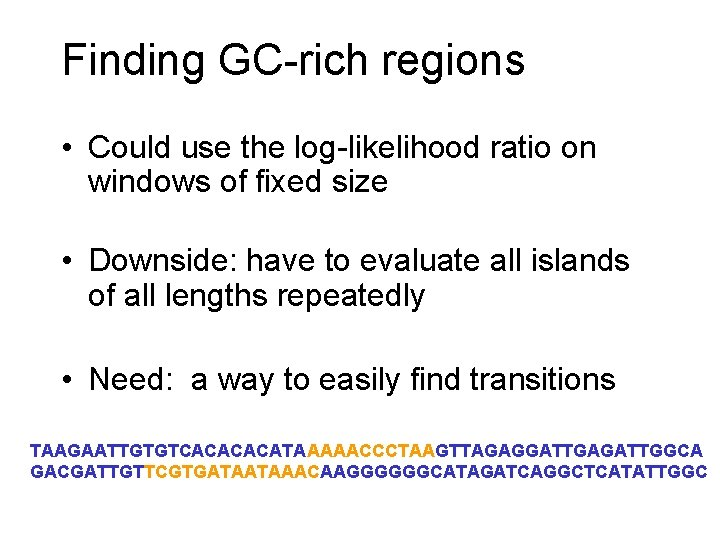Finding GC-rich regions • Could use the log-likelihood ratio on windows of fixed size • Downside: have to evaluate all islands of all lengths repeatedly • Need: a way to easily find transitions TAAGAATTGTGTCACATAAAAACCCTAAGTTAGAGGATTGAGATTGGCA GACGATTGTTCGTGATAATAAACAAGGGGGGCATAGATCAGGCTCATATTGGC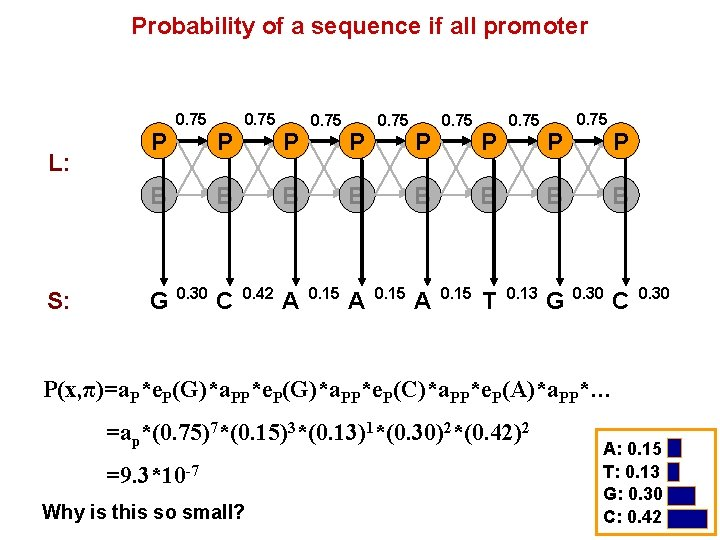Probability of a sequence if all promoter L: P 0. 75 B S: G P 0. 75 B 0. 30 C P 0. 75 B 0. 42 A P 0. 75 B 0. 15 T P 0. 75 B 0. 13 G P B 0. 30 C 0. 30 P(x, π)=a. P*e. P(G)*a. PP*e. P(C)*a. PP*e. P(A)*a. PP*… =ap*(0. 75)7*(0. 15)3*(0. 13)1*(0. 30)2*(0. 42)2 =9. 3*10 -7 Why is this so small? A: 0. 15 T: 0. 13 G: 0. 30 C: 0. 42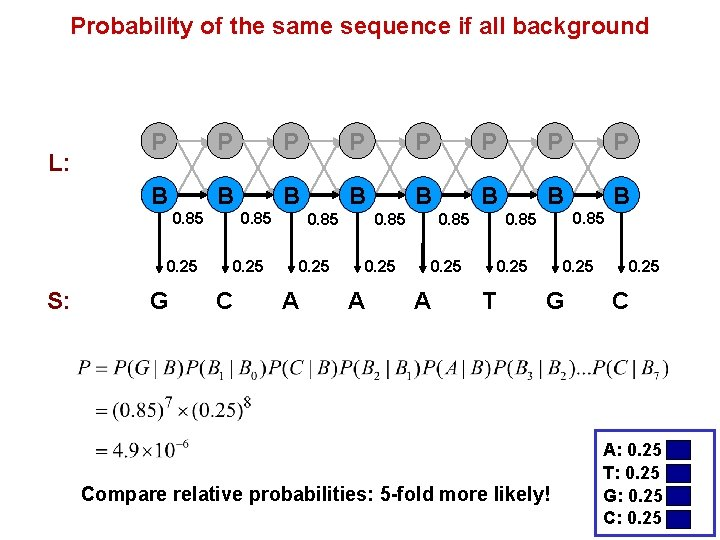Probability of the same sequence if all background L: P P P P B B B B 0. 85 0. 25 S: G 0. 85 0. 25 C 0. 85 0. 25 A 0. 85 0. 25 T 0. 25 G Compare relative probabilities: 5 -fold more likely! 0. 25 C A: 0. 25 T: 0. 25 G: 0. 25 C: 0. 25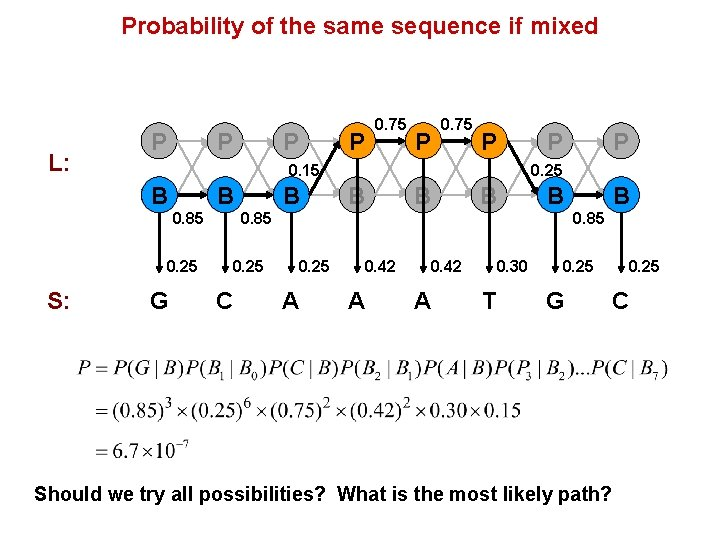Probability of the same sequence if mixed L: P P 0. 75 P 0. 15 B B 0. 85 0. 25 S: P 0. 75 G B P 0. 25 B B 0. 85 0. 25 C P B 0. 85 0. 25 A 0. 42 A 0. 30 T 0. 25 G 0. 25 C Should we try all possibilities? What is the most likely path?The six algorithmic settings for HMMs Learning Decoding Scoring One path 1. Scoring x, one path P(x, π) All paths 2. Scoring x, all paths P(x) = Σπ P(x, π) Prob of a path, emissions Prob of emissions, over all paths 3. Viterbi decoding 4. Posterior decoding π* = argmaxπ P(x, π) π^ = {πi | πi=argmaxk ΣπP(πi=k|x)} Most likely path Path containing the most likely state at any time point. 5. Supervised learning, given π Λ* = argmaxΛ P(x, π|Λ) 6. Unsupervised learning. Λ* = argmaxΛ maxπP(x, π|Λ) Viterbi training, best path 6. Unsupervised learning Λ* = argmaxΛ ΣπP(x, π|Λ) Baum-Welch training, over all paths3. DECODING: What was the sequence of hidden states? Given: Model parameters ei(. ), aij Sequence of emissions x Find: Sequence of hidden states πFinding the optimal path • We can now evaluate any path through hidden states, given the emitted sequences • How do we find the best path? • Optimal substructure! Best path through a given state is: – Best path to previous state – Best transition from previous state to this state – Best path to the end state Viterbi algortithm – Define Vk(i) = Probability of the most likely path through state i=k – Compute Vk(i+1) as a function of maxk’ { Vk’(i) } – Vk(i+1) = ek(xi+1) * maxj ajk Vj(i) Dynamic Programming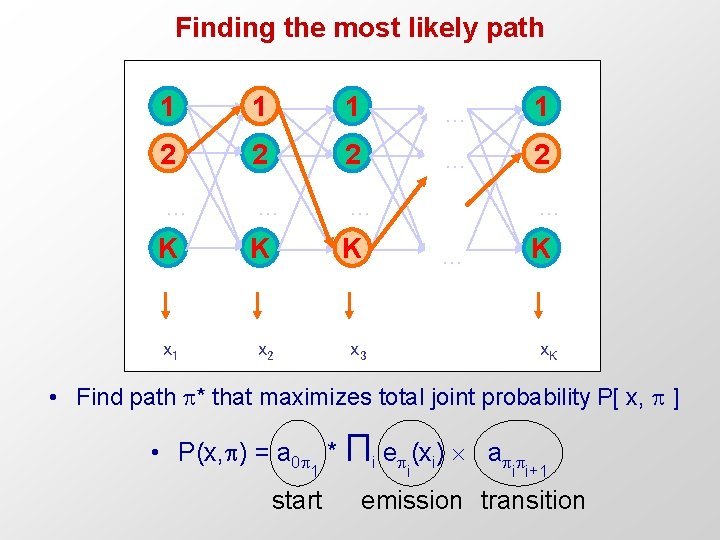Finding the most likely path 1 1 1 … 1 2 2 2 … … … K K K x 1 x 2 x 3 … K … x. K • Find path * that maximizes total joint probability P[ x, ] • P(x, ) = a 0 * Πi e (xi) a 1 start i i i+1 emission transition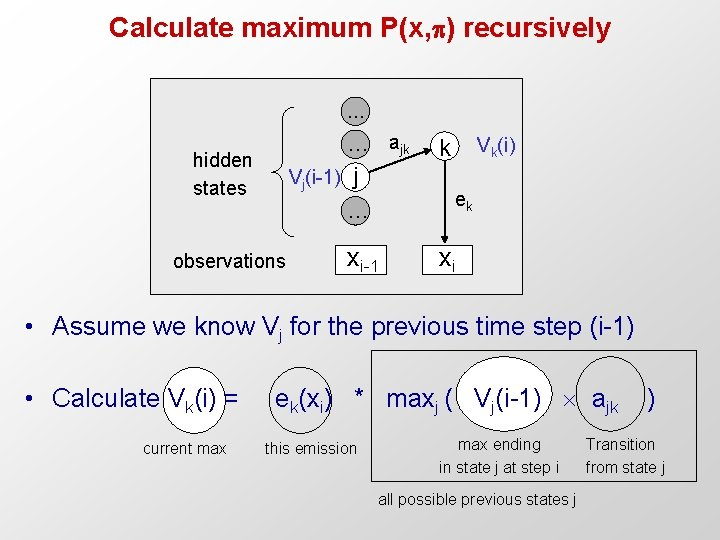Calculate maximum P(x, ) recursively … … ajk Vj(i-1) j … hidden states observations xi-1 Vk(i) k ek xi • Assume we know Vj for the previous time step (i-1) • Calculate Vk(i) = ek(xi) * maxj ( Vj(i-1) ajk ) current max this emission max ending in state j at step i all possible previous states j Transition from state j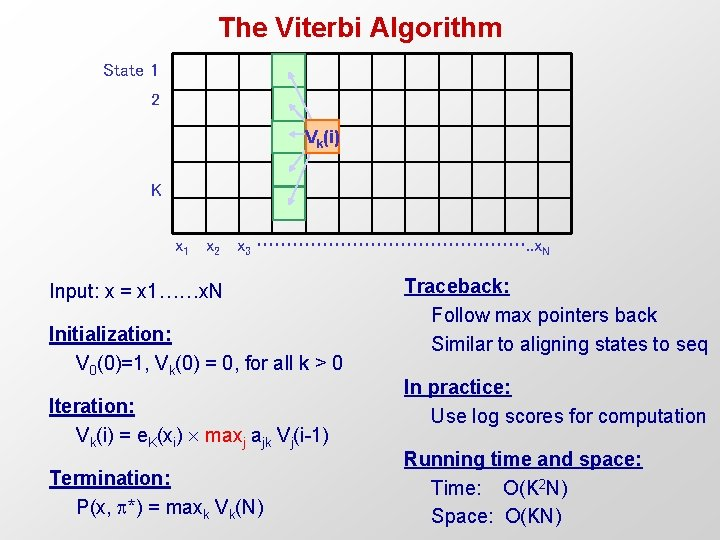The Viterbi Algorithm State 1 2 Vk(i) K x 1 x 2 x 3 ……………………. . x. N Input: x = x 1……x. N Initialization: V 0(0)=1, Vk(0) = 0, for all k > 0 Iteration: Vk(i) = e. K(xi) maxj ajk Vj(i-1) Termination: P(x, *) = maxk Vk(N) Traceback: Follow max pointers back Similar to aligning states to seq In practice: Use log scores for computation Running time and space: Time: O(K 2 N) Space: O(KN)The six algorithmic settings for HMMs Learning Decoding Scoring One path 1. Scoring x, one path P(x, π) All paths 2. Scoring x, all paths P(x) = Σπ P(x, π) Prob of a path, emissions Prob of emissions, over all paths 3. Viterbi decoding 4. Posterior decoding π* = argmaxπ P(x, π) π^ = {πi | πi=argmaxk ΣπP(πi=k|x)} Most likely path Path containing the most likely state at any time point. 5. Supervised learning, given π Λ* = argmaxΛ P(x, π|Λ) 6. Unsupervised learning. Λ* = argmaxΛ maxπP(x, π|Λ) Viterbi training, best path 6. Unsupervised learning Λ* = argmaxΛ ΣπP(x, π|Λ) Baum-Welch training, over all paths2. EVALUATION (how well does our model capture the world) Given: Model parameters ei(. ), aij Sequence of emissions x Find: P(x|M), summed over all possible paths π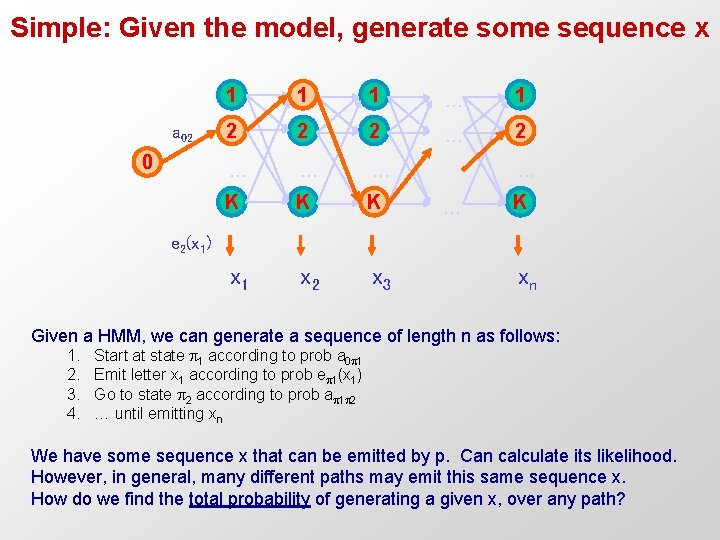Simple: Given the model, generate some sequence x a 02 0 1 1 1 … 1 2 2 2 … … … K K K x 1 x 2 x 3 … … K e 2(x 1) xn Given a HMM, we can generate a sequence of length n as follows: 1. 2. 3. 4. Start at state 1 according to prob a 0 1 Emit letter x 1 according to prob e 1(x 1) Go to state 2 according to prob a 1 2 … until emitting xn We have some sequence x that can be emitted by p. Can calculate its likelihood. However, in general, many different paths may emit this same sequence x. How do we find the total probability of generating a given x, over any path?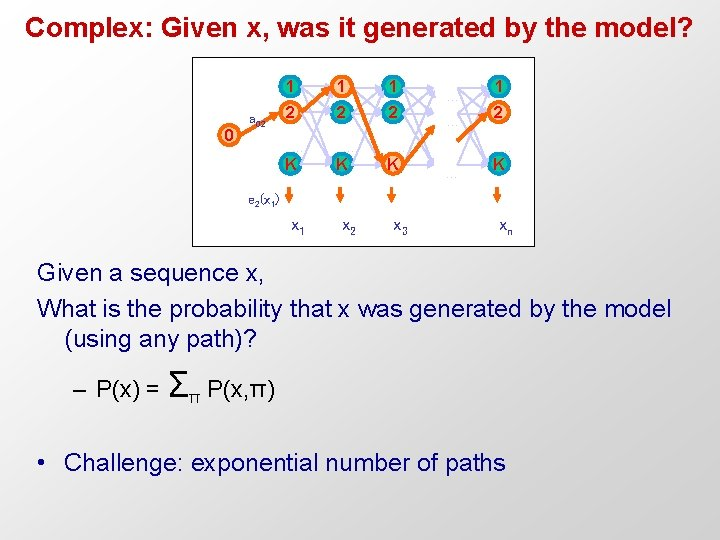Complex: Given x, was it generated by the model? 0 a 02 1 1 1 2 2 2 … K … … … K 1 2 … … K e 2(x 1) x 1 x 2 x 3 xn Given a sequence x, What is the probability that x was generated by the model (using any path)? – P(x) = Σπ P(x, π) • Challenge: exponential number of pathsCalculate probability of emission over all paths • Each path has associated probability – Some paths are likely, others unlikely: sum them all up Return total probability that emissions are observed, summed over all paths – Viterbi path is the most likely one • How much ‘probability mass’ does it contain? • (cheap) alternative: – Calculate probability over maximum (Viterbi) path π* – Good approximation if Viterbi has highest density – BUT: incorrect • (real) solution – Calculate the exact sum iteratively • P(x) = Σπ P(x, π) – Can use dynamic programmingThe Forward Algorithm – derivation Define the forward probability: fl(i) = P(x 1…xi, i = l) = 1… i-1 P(x 1…xi-1, 1, …, i-2, i-1, i = l) el(xi) = k 1… i-2 P(x 1…xi-1, 1, …, i-2, i-1=k) akl el(xi) = k fk(i-1) akl el(xi) = el(xi) k fk(i-1) akl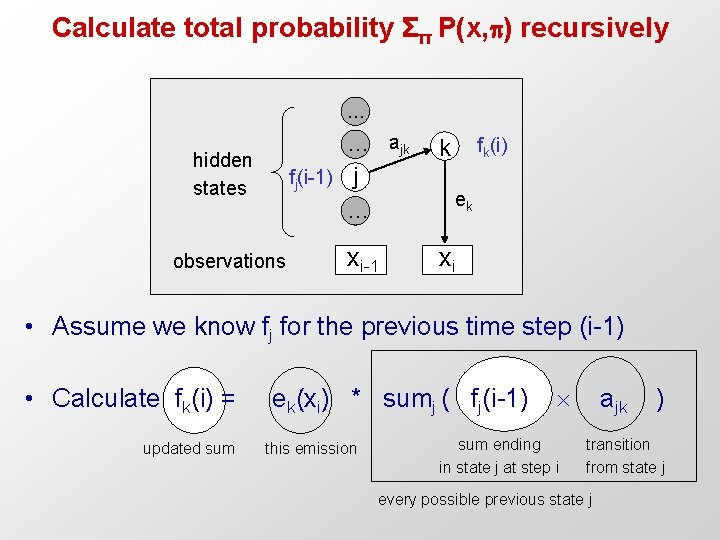Calculate total probability Σπ P(x, ) recursively … … ajk fj(i-1) j … hidden states observations xi-1 fk(i) k ek xi • Assume we know fj for the previous time step (i-1) • Calculate fk(i) = ek(xi) * sumj ( fj(i-1) ajk ) updated sum this emission sum ending in state j at step i transition from state j every possible previous state jThe Forward Algorithm State 1 2 fk(i) K x 1 x 2 x 3 ……………………. . x. N Input: x = x 1……x. N Initialization: f 0(0)=1, fk(0) = 0, for all k > 0 Iteration: fk(i) = e. K(xi) sumj ajk fj(i-1) Termination: P(x, *) = sumk fk(N) In practice: Sum of log scores is difficult approximate exp(1+p+q) scaling of probabilities Running time and space: Time: O(K 2 N) Space: O(KN)The six algorithmic settings for HMMs Learning Decoding Scoring One path 1. Scoring x, one path P(x, π) All paths 2. Scoring x, all paths P(x) = Σπ P(x, π) Prob of a path, emissions Prob of emissions, over all paths 3. Viterbi decoding 4. Posterior decoding π* = argmaxπ P(x, π) π^ = {πi | πi=argmaxk ΣπP(πi=k|x)} Most likely path Path containing the most likely state at any time point. 5. Supervised learning, given π Λ* = argmaxΛ P(x, π|Λ) 6. Unsupervised learning. Λ* = argmaxΛ maxπP(x, π|Λ) Viterbi training, best path 6. Unsupervised learning Λ* = argmaxΛ ΣπP(x, π|Λ) Baum-Welch training, over all pathsIntroducing memory • State, emissions, only depend on current state • How do you count di-nucleotide frequencies? – Cp. G islands – Codon triplets – Di-codon frequencies • Introducing memory to the system – Expanding the number of statesExample 2: Cp. G islands: incorporating memory a. AT A+ T+ a. AC A+ C+ G+ T+ A: 1 C: 0 G: 0 T: 0 A: 0 C: 1 G: 0 T: 0 A: 0 C: 0 G: 1 T: 0 A: 0 C: 0 G: 0 T: 1 a. GT C+ a. GC G+ • Markov Chain – Q: states – p: initial state probabilities – A: transition probabilities • HMM – Q: states – V: observations – p: initial state probabilities – A: transition probabilities – E: emission probabilities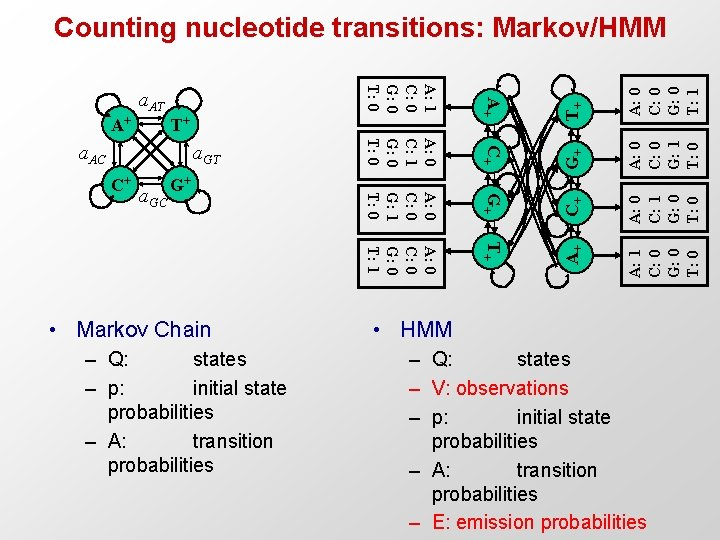G+ C+ A: 0 C: 1 G: 0 T+ A: 1 C: 0 G: 0 T: 0 a. GC G+ • Markov Chain – Q: states – p: initial state probabilities – A: transition probabilities A: 0 C: 0 G: 1 T: 0 G+ A: 0 C: 0 G: 0 T: 1 C+ A: 0 C: 0 G: 0 T: 1 a. GT T+ A+ A: 0 C: 0 G: 1 T: 0 T+ a. AC C+ A: 0 C: 1 G: 0 T: 0 A+ A: 1 C: 0 G: 0 T: 0 a. AT A+ Counting nucleotide transitions: Markov/HMM • HMM – Q: states – V: observations – p: initial state probabilities – A: transition probabilities – E: emission probabilities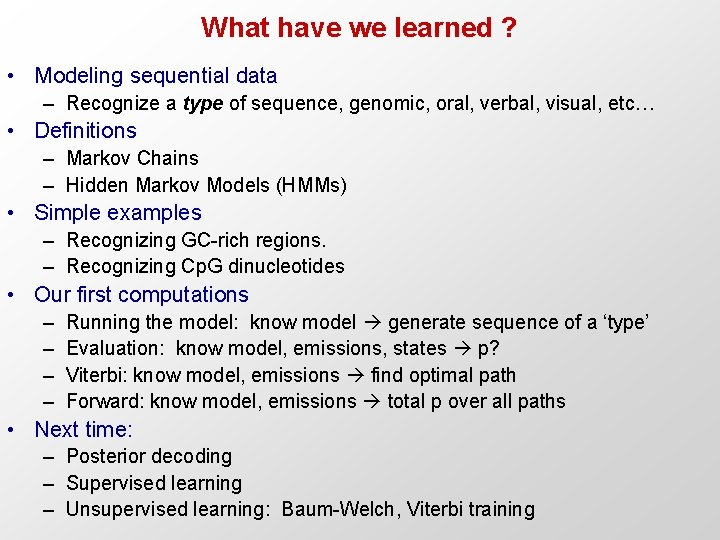What have we learned ? • Modeling sequential data – Recognize a type of sequence, genomic, oral, verbal, visual, etc… • Definitions – Markov Chains – Hidden Markov Models (HMMs) • Simple examples – Recognizing GC-rich regions. – Recognizing Cp. G dinucleotides • Our first computations – – Running the model: know model generate sequence of a ‘type’ Evaluation: know model, emissions, states p? Viterbi: know model, emissions find optimal path Forward: know model, emissions total p over all paths • Next time: – Posterior decoding – Supervised learning – Unsupervised learning: Baum-Welch, Viterbi training